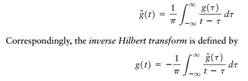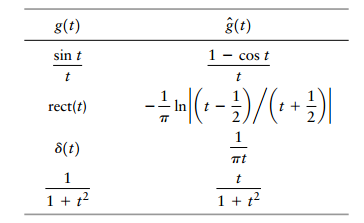Create an Account

Home / Questions / The Hilbert transform of a Fourier transformable signal gt is defined by Using these tw...

The Hilbert transform of a Fourier transformable signal gt is defined by Using these two formulas derive the following set of Hilbert transform pairs

The Hilbert transform of a Fourier transformable signal g(t) is defined byUsing these two formulas, derive the following set of Hilbert-transform pairs:Jul 31 2020 View more View LessSubscribe To Get Solution